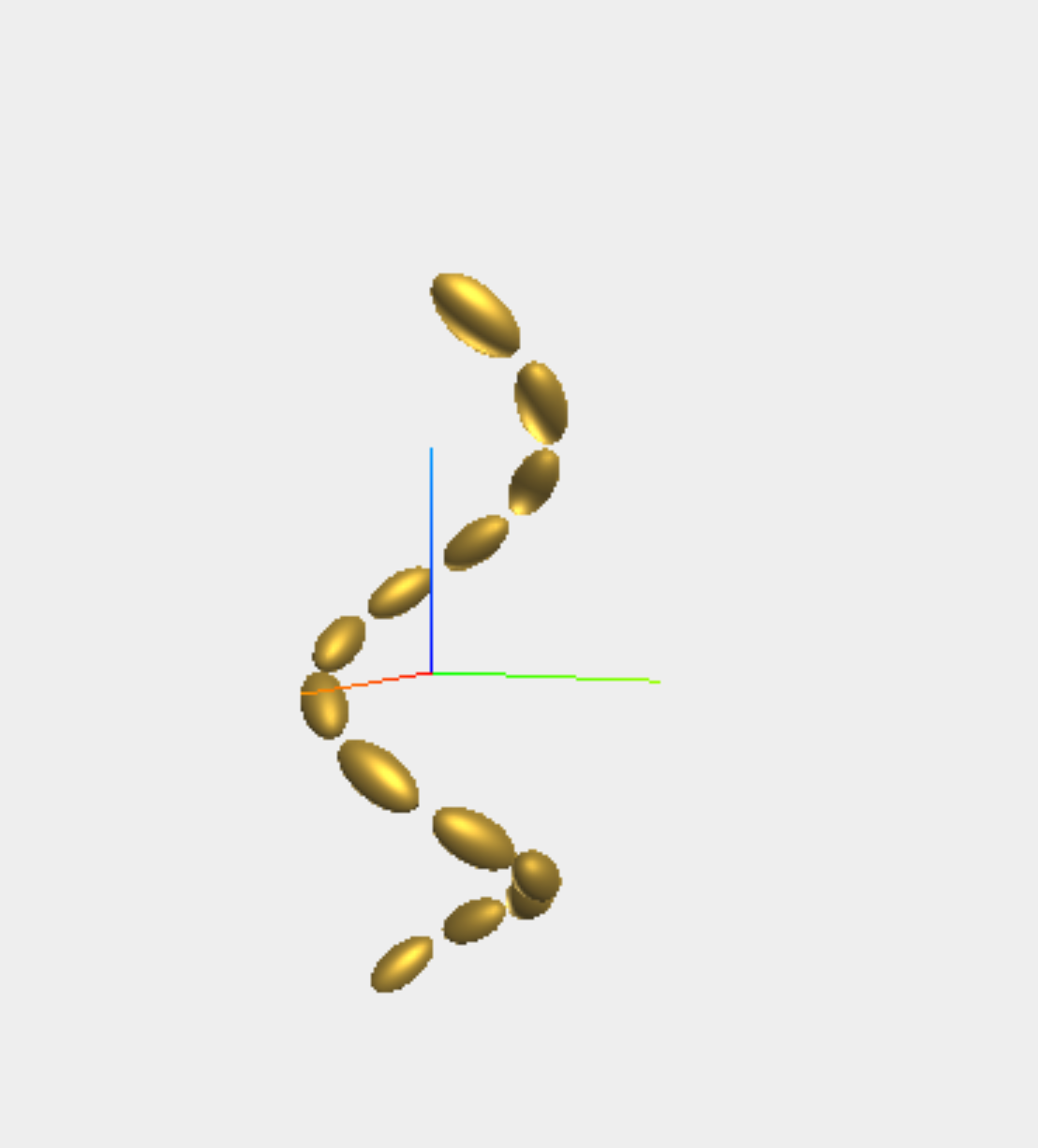# Cluster geometries

CoupledDipole.cluster_singleFunction
cluster_single(a::T, b::T, c::T, α::T = 0.0, β::T = 0.0, γ::T = 0.0) where T <: Real

Particle cluster consisting of a single particle at the origin

• a,b,c: semi-axis along x,y,z
• α,β,γ: Euler angles
• material: String referencing the material of the particle
• type: String, "point" dipole or "particle"

Examples

cluster_single(1.0,2.0,3.0)
source
CoupledDipole.cluster_dimerFunction
cluster_dimer(d::T, a::T, b::T, c::T, ϕ::T = 0.0, α_1::T = 0.0, α_2::T = 0.0) where T <: Real

Particle cluster consisting of 2 identical particles separated along y

• a,b,c: semi-axes along x,y,z
• ϕ: dihedral angle between both particles seen along the y-axis
• α_1,α_2: angle of each particle with the y axis
• material: String referencing the material of every particle
• type: String, "point" dipole or "particle"

Examples

cluster_dimer(80, 10, 10, 20)
sourceCoupledDipole.cluster_helixFunction
cluster_helix(N, a, b, c, R, Λ, δ = π/4, δ_0 = 0, handedness="left",
material = "Au", type="particle")

Helical cluster of N identical particles with axis along z

• N: number of particles
• a,b,c: semi-axes along x,y,z
• R: helix radius
• Λ: helix pitch
• δ: angle between subsequent particles
• δ: starting angle
• handedness: "left" or "right"
• material: String referencing the material of every particle
• type: String, "point" dipole or "particle"

Examples

cluster_helix(4, 20, 20, 30, 50, 300)
source
CoupledDipole.cluster_lineFunction
cluster_line(N, Λ, a, b, c, φ, θ, ψ, material = "Au", type="particle")

Line of N identical particles in the x direction

• N: number of particles (approximate if not exact square)
• a,b,c: semi-axes along x,y,z
• Λ: array pitch
• φ, θ, ψ: particle Euler angles
• material: String referencing the material of every particle
• type: String, "point" dipole or "particle"

Examples

cluster_line(3, 500, 20, 20, 30, 0, 0, 0)
source
CoupledDipole.cluster_arrayFunction
cluster_array(N, Λ, a, b, c, φ, θ, ψ, material = "Au", type="particle")

Square array of N identical particles in the xy plane

• N: number of particles (approximate if not exact square)
• a,b,c: semi-axes along x,y,z
• Λ: array pitch
• φ, θ, ψ: particle Euler angles
• material: String referencing the material of every particle
• type: String, "point" dipole or "particle"

Examples

cluster_array(4, 500, 20, 20, 30, 0, 0, 0)
source